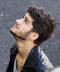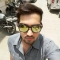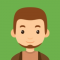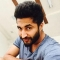## Check if the String contains only unicode letters or digits in JavaPublished on 07-Jan-2019 11:56:42
To check whether a String contains only unicode letters or digits in Java, we use the isLetterOrDigit() method and charAt() method with decision-making statements.The isLetterOrDigit(char ch) method determines whether the specific character (Unicode ch) is either a letter or a digit. It returns a boolean value, either true or false.Declaration ... Read More

## Get the base 10 logarithm of a value in JavaPublished on 07-Jan-2019 11:52:34
To get the base 10 logarithm of value in Java, we use the java.lang.Math.log10() method. This method returns the log of value to the base 10 in the form of a double. If a number is 10n, then the result is n. If the value passed is NaN or negative, ... Read More

## Get the hyperbolic sine of a given value in JavaPublished on 07-Jan-2019 11:48:51
In order to get the hyperbolic sine of a given value in Java, we use the java.lang.Math.sinh() method. The sinh() method accepts an argument in radians and returns the hyperbolic sine of the argument which acts as the angle.Declaration −  The java.lang.Math.sinh() is declared as follows −public static double sinh(double ... Read More

## Get the hyperbolic cosine of a given value in JavaPublished on 07-Jan-2019 11:44:44
In order to get the hyperbolic cosine of a given value in Java, we use the java.lang.Math.cosh() method. The cosh() method accepts an argument in radians and returns the hyperbolic cosine of the argument which acts as the angle.Declaration − - The java.lang.Math.cosh() is declared as follows −public static double ... Read More

## Round float and double numbers in JavaPublished on 07-Jan-2019 11:39:55
In order to round float and double numbers in Java, we use the java.lang.Math.round() method. The method accepts either double or float values and returns an integer value. It returns the closest integer to number. This is computed by adding ½ to the number and then flooring it.Declaration - The ... Read More

## Get square root of a number using Math.sqrt in JavaPublished on 07-Jan-2019 11:37:02
In order to get the square root of a number in Java, we use the java.lang.Math.pow() method. The Math.pow(double a) method accepts one argument of the double data type and returns a value which is the square root of the argument.Declaration − The java.lang.Math.sqrt() method is declared as follows −public ... Read More

## Get power using Math.pow in JavaPublished on 07-Jan-2019 11:32:09
In order to get the power of a number in Java, we use the java.lang.Math.pow() method. The Math.pow(double a, double b) method accepts two arguments of the double data type and returns a value of the first parameter raised to the power of the second argument.Declaration - The java.lang.Math.pow() method ... Read MorePublished on 07-Jan-2019 11:25:39
To obtain the natural log of a number, we use the java.lang.Math.log() method. The Math.log() method returns the natural logarithm i.e. log to the base e of a double value. If the value passed is NaN or negative, the result is NaN. If the value passed is positive infinity, then ... Read More

## Get the maximum of two numbers using Math.max in JavaPublished on 07-Jan-2019 11:19:59
To obtain the maximum of two numbers using Math.max in Java, we use the java.lang.Math.max() method. The Math.max() accepts two numbers and returns the greater of the two. The result is closer to positive infinity on the number line. Even if one of the values is not a number(NaN), the ... Read More

## Get floor value of a number using Math.floor in JavaPublished on 07-Jan-2019 11:15:07
To get the floor value of a number, we use the java.lang.Math.floor() method. The Math.floor() method returns the largest (closest to positive infinity) double value which is less than or equal to the parameter and has a value which is equal to a mathematical integer on the number line. If ... Read More